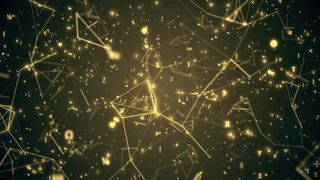# 'Imaginary' numbers are real (sort of)Imaginary numbers have a real physical meaning, according to a new set of studies.

Imaginary numbers, which can be combined with real numbers to form complex numbers, are numbers that were thought not to have any sort of analogue in daily life. Real numbers, by contrast, are clearly observable: 1 or 2 is easy enough to recognize in the real world; pi is the ratio of a circle's circumference to its diameter; 32 degrees Fahrenheit (0 degrees Celsius) is the freezing point of water. But there's nothing in the real world that can represent an imaginary number like the square root of negative 1.

Until now, perhaps: New research, conducted by a team led by Alexander Streltsov of the University of Warsaw in Poland and Kang-Da Wu of the University of Science and Technology of China in Hefei, finds that imaginary numbers actually carry real information about quantum states.

"They are not a mere mathematical artifact," said study co-author Carlo Maria Scandolo, a mathematical physicist at the University of Calgary in Canada. Instead, he said, "complex numbers really do exist."

Imaginary numbers have always had a place in quantum theory. The equations used to describe the behavior of tiny quantum particles are expressed with these complex numbers. This raised a question, Scandolo told Live Science: Are these numbers just mathematical tools, or do they represent something real about the quantum states these equations describe?

To find out, the researchers used a mathematical framework to determine if imaginary numbers are  a "resource." In quantum theory, "resource" has a specific meaning: a property that enables new actions that would otherwise be impossible. Quantum entanglement is a resource in quantum theory, because it allows actions such as quantum teleportation, or the transfer of information between locations.

If imaginary numbers are a resource, they'd enable physicists to do more than they could if imaginary numbers weren't present. The team's calculations suggested that imaginary numbers are indeed a resource. But the next step was to check that math in the real world.

To do so, the researchers set up an optics experiment in which a source sent entangled photons (particles of light) to two receivers, "Alice" and "Bob." The goal was for Alice and Bob to determine the quantum states of the photons. They could perform local measurements on their own photons and then compare the measurements, which would allow Alice and Bob to calculate their probability of guessing the correct state for the opposite photon.

For some pairs of quantum states, the researchers found, Alice and Bob could guess the states with 100% accuracy — but only if they were allowed to use imaginary numbers in their local measurements. When they were forbidden from using imaginary numbers, it became impossible to accurately tell the two states apart.

"If I remove complex numbers, in these cases, I completely lose my ability to distinguish these two states," Scandolo said.

In other words, the experiment found the same thing as the math: The loss of complex numbers equaled the loss of real information about a quantum system.

The information these complex numbers carry isn't related to a simple physical property, like the spin of an electron. Instead, Scandolo said, it has to do with the ability to extract information from a particle where this particle is located, without considering interactions with other particles at a distance.

The researchers now plan to expand their search for other situations in quantum theory in which imaginary numbers might be a quantum resource. They also want to find out more about how imaginary numbers play a role in situations in which using quantum information is advantageous. For example, the information carried by imaginary numbers might also help explain the underlying reasons why quantum computing allows for actions that traditional computing doesn't, Scandolo said.

"It's important both from a foundational point of view but also as a way of understanding how we can better harness quantum resources and how the quantum world works," he said.

The research was published March 1 in the journals Physical Review A and Physical Review Letters

Originally published on Live Science.